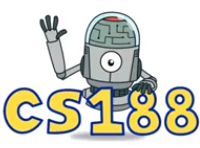# CS 61B Resources

## By Allen Guo

Last Updated: 04/25/2018

### General Resources

• CS 61B course website: link.
• Exams from past semesters: link.

### Java

• Java syntax practice problems: link.
• OpenJDK source, i.e., how Java built-in classes are implemented: link.
• For example, here's how Java's real HashMap is implemented: link.
• "Java is Pass-by-Value, Dammit!" by Scott Stanchfield.
• A quick guide to method selection: link.

### Git

• Git is the name of the version control software. It's free and open source. GitHub is a company that hosts Git repositories (repos). You can use it as a central Git server for your projects.
• How to fix common Git problems: link.
• Make your shell Git-friendly with autocompletion and prompt support.
• I have custom Bash aliases for Git commands, which you can see in the "Git" section here: link.
• "A Hacker's Guide to Git" by Joseph Wynn.

### Asymptotics

• The single most confusing topic in asymptotics is the difference between best-case/worst-case and big-O/Theta/Omega.
• Big-O/Theta/Omega describes the runtime of an algorithm in one or multiple cases. Best-case/worst-case describes a particular input case for an algorithm.
• Here's an example: "The worst-case runtime for quicksort is Theta(n^2)."
• Notice how "worst-case" describes the input. For example, for quicksort where the first element is chosen as the pivot, the worst-case input is an already-sorted list.
• What's confusing is that I could also say, "The runtime for quicksort is O(n^2)."
• This is a more general statement: I'm implying that this statement is true for all cases/inputs.
• Notice that this does not imply that the worst-case runtime of quicksort is Theta(n^2) (why not?).
• You can't perform asymptotic analysis just by blindly following rules like "multiply for nested loops".
• Common sums:
• Sum of numbers: 1 + 2 + 3 + 4 + ... + n = Theta(n^2).
• Sum of squares: 1 + 4 + 9 + 16 + ... + n^2 = Theta(n^3).
• Geometric sum: 1 + 2 + 4 + 8 + ... + n = Theta(n).
• Uncommon sums:
• Sum of logs: log(1) + log(2) + log(3) + log(4) + ... + log(n) = log(n!) = Theta(n log n).
• Sum of subarray lengths: (n)(1) + (n-1)(2) + ... + (2)(n-1) + (1)(n) = Theta(n^3).

### BSTs

• Some problems:
• Write a method that, given a binary tree of integers, returns if the tree is a valid BST. (Solution: link.)
• Write a method that, given a BST and two nodes in that BST, returns the lowest common ancestor of those two nodes, i.e., the common ancestor of the nodes farthest from the root. (Solution: link.)

### 2-3 Trees (and LLRB Trees)

• Diagram with examples of insertion: link.
• Visualizer: link (use "Max. Degree = 3" for 2-3 tree).

### Heaps

• Students tend to confuse heaps and BSTs. Do you know what the differences are?
• Proof that bottom-up heapify takes linear time: link (see the "BuildHeap Analysis" section).

### Graphs

• CS 188 exam prep worksheet: link. Treat "Uniform Cost Search" as a synonym for Dijkstra's algorithm. Ignore problems about "Greedy Search".
• Solutions are here.

### Dynamic Programming (DP)

• DP isn't a specific algorithm, but rather a general problem-solving technique.
• So learning DP is less like learning Dijkstra's and more like learning recursion.
• Don't worry if the definition of DP is confusing at first.
• Do practice problems. Then think about what those problems had in common. Then go back to the definition.
• Try to solve the problem yourself before looking at the answer.

### Inspiration and Wisdom### Internships and Interviews

• If you have any interest at all in working in software development in the future, I highly recommend you apply for internships this cycle, even if you don't feel ready.
• At the very least, you'll get a feel for what the process is like and what employers are looking for.
• "A Brief Guide to Tech Internships" by Alexey Komissarouk.
• Except don't wait until winter break to start applying. Lots of positions at popular companies fill up by the end of fall semester.
• For interview practice, I (like everyone else) recommend Cracking the Coding Interview.
• Find a buddy, reserve a library room with a whiteboard, and take turns interviewing each other.
• Study the problem-solving flowchart from the book: link.
• Tip: Keep a list of interesting technical questions that you've encountered, including the solutions.
• Review the list often and add to it after every practice session.
• Before every real interview, go through the entire list and make sure you know everything on it.
• Coding interview tips from Interview Cake: link.
• A list of coding questions by Max Noy: link.
• An even bigger list by Program Creek: link.
• A still bigger list by Vivek Srivastava: link.

### What Courses Do I Take Next?• CS draft schedule (i.e., who's teaching what): link.